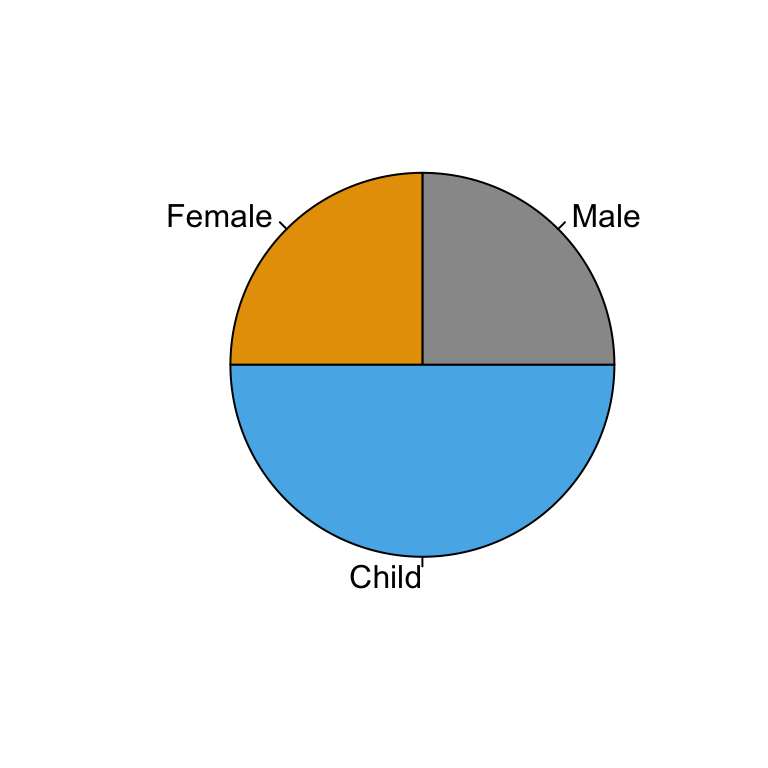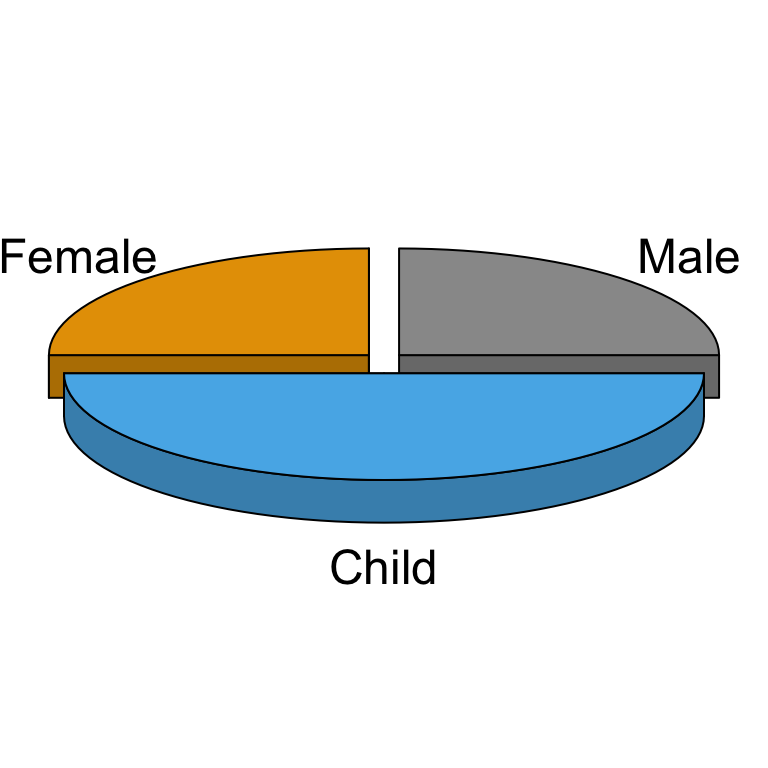# Pie Charts - R Base Graphs

Previously, we described the essentials of R programming and provided quick start guides for importing data into R.

Here, we’ll describe how to create pie charts in R. The R base function pie() can be used for this.

1. Launch RStudio as described here: Running RStudio and setting up your working directory

2. Prepare your data as described here: Best practices for preparing your data and save it in an external .txt tab or .csv files

3. Import your data into R as described here: Fast reading of data from txt|csv files into R: readr package.

# Create some data

``````df <- data.frame(
group = c("Male", "Female", "Child"),
value = c(25, 25, 50)
)
df``````
``````##    group value
## 1   Male    25
## 2 Female    25
## 3  Child    50``````

# Create basic pie charts: pie()

The function pie() can be used to draw a pie chart.

``pie(x, labels = names(x), radius = 0.8)``

• x: a vector of non-negative numerical quantities. The values in x are displayed as the areas of pie slices.
• labels: character strings giving names for the slices.
• radius: radius of the pie circle. If the character strings labeling the slices are long it may be necessary to use a smaller radius.

``pie(df\$value, labels = df\$group, radius = 1)````````# Change colors
pie(df\$value, labels = df\$group, radius = 1,
col = c("#999999", "#E69F00", "#56B4E9"))``````# Create 3D pie charts: plotix::pie3D()

Te function pie3D()[in plotrix package] can be used to draw a 3D pie chart.

Install plotrix package:

``install.packages("plotrix")``

Use pie3D():

``````# 3D pie chart
library("plotrix")
pie3D(df\$value, labels = df\$group, radius = 1.5,
col = c("#999999", "#E69F00", "#56B4E9"))````````````# Explode the pie chart
pie3D(df\$value, labels = df\$group, radius = 1.5,
col = c("#999999", "#E69F00", "#56B4E9"),
explode = 0.1)``````# Infos

This analysis has been performed using R statistical software (ver. 3.2.4).